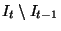Next: Results Up: Role Correlation Previous: Challenges

## The Role Correlation Algorithm

The correlation algorithm operates by comparing the results of two executions of the grouping algorithms. Let Pt-1 and Pt be the group sets generated by the grouping algorithm at time t-1 and t respectively. The correlation algorithm updates the ID set of Pt, so that IDGt = IDGt-1, whereand, if and only if Gt and Gt-1 considered to represent the same logical role. More specifically, the connection patterns of the members of Gt and those of Gt-1 are very similar. The groups correlation algorithm correlates the IDGt and IDGt-1 in a meaningful manner and thus allow applications to preserve data specific to a particular group.

The role correlation algorithm:

• Isolates the primary events, such as node arrivals and removals, that directly affects the connection habits of groups,
• Identifies nodes that have not changed their neighbors,
• Heuristically computes the time-varying similarity between the connection habits of two groups formed at times t and t-1, and assigns IDGt = IDGt-1 if and only if the role of hosts (in terms on their connection patterns) in Gt-1 can be considered identical to the role of hosts in Gt.

First, the correlation algorithm eliminates the differences between the two host sets, It and It-1, so that it can compare the connection patterns meaningfully. The algorithm computes the set of nodes that existed at time t-1 but have been removed in time t (), and the set of nodes that only appear at time t (). All new nodes are removed from It and deleted nodes are removed from It-1. Thus, the changes in connection set of each host is only as a direct result of changing connection patterns between the host and its neighbors (which existed at time t).

Second, the algorithm heuristically identifies the set,, of nodes that are very like to play the same logical roles from t-1 to t. We say that the two nodes ht and ht-1 are highly likely to be the same machine (i.e. it hasn't changed its logical role) if they have the identical connection sets. Specifically,. We will explain shortly how we use the fact that a hostto our advantage in computing the time varying similarity measure.

The role correlation algorithm will determine whether the two groups Gt and Gt-1 are the same group by heuristically computing the time-varying similarity measure and comparing against the pre-defined threshold. The group correlation algorithm operates as follows:

1. For each group Gt, identify Gt and Gt-1 as the same group if i) Gt-1 has the strongest time-varying similarity with Gt, among all the groups in Pt-1 and ii) the average number of connections is at least within Thi percent of the average number of connections of Gt-1.

2. For each group pair (Gt, Gt-1) that remain uncorrelated, decide whether Gt and Gt-1 represent the same logical group based on how similar the connection patterns between Gt and its neighbor groups are to those between Gt-1 and its neighbor groups.

Step 1 decides whether the two groups Gt and Gt-1 are identical based on the time varying similarity measure. As in Section 4.2, we compute the similarity measure based on the average number of connections between the groups and their common neighbors. However, finding the common neighbor set between Gt and Gt-1 is not trivial. This is because we cannot simply assume that a neighborand a neighborare the same host even if they have the same host identifier. We use the following techniques to identify the common neighbor set:

• If a neighbor ht of Gt shares the same host identifier with the neighbor ht-1 of Gt-1 and both have been considered highly likely to be the same host (i.e.), we assume ht is the neighbor to Gt in the same way as ht-1 is to Gt-1.

• For each neighbor pairs (ht, ht-1) that are not considered as highly likely to be the same host, we assume ht is the neighbor to Gt in the same way as ht-1 is to Gt-1 if and only if the following condition is true. The connection set size of ht-1 is within Thi percent of that of ht and no other neighbor of Gt-1 has the connection set size closer to that of ht.
The algorithm then computes the time-varying similarity measure between each neighbor pair (ht, ht-1), which meets the aforementioned requirements, as the minimum of the average number of connections between ht and Gt and between ht-1 and Gt-1. If the sum of the similarity measures for all common neighbor pairs within the bounds of the specified thresholds , the algorithm declares that groups Gt and Gt-1 mean the same group.Next: Results Up: Role Correlation Previous: Challenges
Godfrey Tan 2003-04-01